# Why use dollar sign (\$) in Excel formulas - absolute and relative cell references

When writing an Excel formula, \$ in cell references confuses many users. But the explanation is very simple. The dollar sign in an Excel cell reference serves just one purpose - it tells Excel whether to change or not to change the reference when the formula is copied to other cells. And this short tutorial provides full details about this great feature.

The importance of Excel cell reference can hardly be overstated. Get the insight into the difference between absolute, relative and mixed references, and you are halfway to mastering the power and versatility of Excel formulas and functions.

All of you have probably seen the dollar sign (\$) in Excel formulas and wondered what's that all about. Indeed, you can reference one and the same cell in four different ways, for example A1, \$A\$1, \$A1, and A\$1.

The dollar sign in an Excel cell reference affects just one thing - it instructs Excel how to treat the reference when the formula is moved or copied to other cells. In a nutshell, using the \$ sign before the row and column coordinates makes an absolute cell reference that won't change. Without the \$ sign, the reference is relative and it will change.

If you are writing a formula for a single cell, you can go with any reference type and get the formula right anyway. But if you intend to copy your formula to other cells, choosing the appropriate cell reference type is crucial.  If you feel lucky, you can toss a coin :) If you want to be serious, then invest a few minutes in learning the ins-and-outs of absolute and relative cell references in Excel, and when to use which one.

## What is an Excel cell reference?

To put it simply, a cell reference in Excel is a cell address. It tells Microsoft Excel where to look for the value you want to use in the formula.

For example, if you enter a simple formula =A1 in cell C1, Excel will pull a value from cell A1 into C1: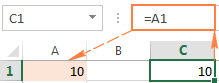As already mentioned, as long as you write a formula for a single cell, you are free to use any reference type, with or without the dollar sign (\$), the result will be the same: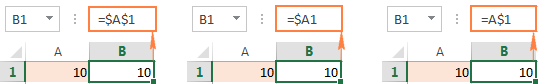But if you want to move or copy the formula across the worksheet, it's very important that you choose the right reference type for the formula to get copied correctly to other cells. The following sections provide the detailed explanation and formula examples for each cell reference type.

Note. Apart from the A1 reference style, where columns are defined by letters and rows by numbers, there also exist the R1C1 reference style where both rows and columns are identified by numbers (R1C1 designates row 1, column 1).

Because A1 is the default reference style in Excel and it is used most of the time, we will discuss only the A1 type references in this tutorial. If someone is currently using the R1C1 style, you can turn it off by clicking File > Options > Formulas, and then unchecking the R1C1 reference style box.

## Excel relative cell reference (without \$ sign)

A relative reference in Excel is a cell address without the \$ sign in the row and column coordinates, like A1.

When a formula with relative cell references in copied to another cell, the reference changes based on a relative position of rows and columns. By default, all references in Excel are relative. The following example shows how relative references work.

Supposing you have the following formula in cell B1:

`=A1*10`

If you copy this formula to another row in the same column, say to cell B2, the formula will adjust for row 2 (A2*10) because Excel assumes you want to multiply a value in each row of column A by 10.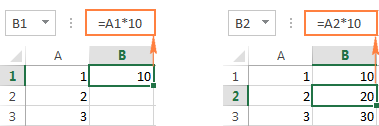If you copy the formula with a relative cell reference to another column in the same row, Excel will change the column reference accordingly: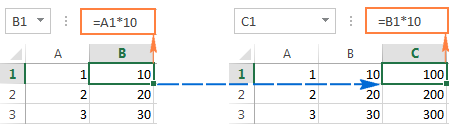And if you copy or move an Excel formula with a relative cell reference to another row and another column, both column and row references will change: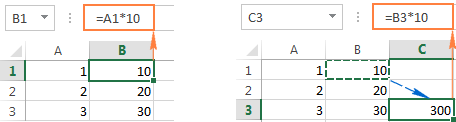As you see, using relative cell references in Excel formulas is a very convenient way to perform the same calculations across the entire worksheet. To better illustrate this, let's discuss a real-life example.

#### Using relative reference is Excel - formula example

Supposing you have a column of USD prices (column B) in your worksheet, and you want to convert them to EUR. Knowing the USD - EUR conversion rate (0.93 at the moment of writing), the formula for row 2, is as simple as `=B2*0.93`. Notice, that we are using an Excel relative cell reference, without the dollar sign.

Pressing the Enter key will get the formula calculated, and the result will immediately appear in the cell.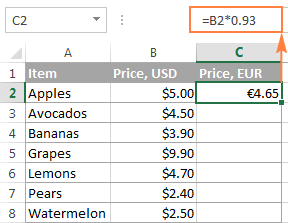Tip. By default, all cell references in Excel are relative references. So, when writing a formula, you can add a relative reference by clicking the corresponding cell on the worksheet instead of typing a cell reference manually.

To copy the formula down the column, hover the mouse over the fill handle (a small square in the bottom-right corner of the selected cell). As you do this, the cursor will change to a thin black cross, and you hold and drag it over the cells you want to auto-fill.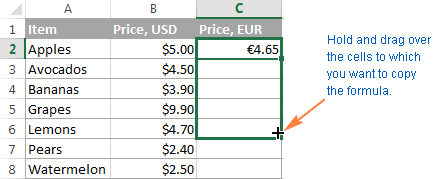That's it! The formula is copied to other cells with relative references that are adjusted properly for each individual cell. To make sure that a value in each cell is calculated correctly, select any of the cells and view the formula in the formula bar. In this example, I've selected cell C4, and see that the cell reference in the formula is relative to row 4, exactly as it should be: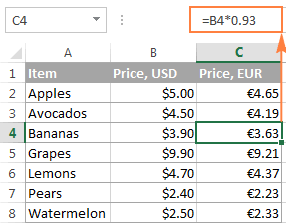## Excel absolute cell reference (with \$ sign)

An absolute reference in Excel is a cell address with the dollar sign (\$) in the row or column coordinates, like \$A\$1.

The dollar sign fixes the reference to a given cell, so that it remains unchanged no matter where the formula moves. In other words, using \$ in cell references allows you to copy the formula in Excel without changing references.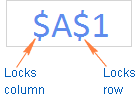For example, if you have 10 in cell A1 and you use an absolute cell reference (\$A\$1), the formula `=\$A\$1+5` will always return 15, no matter what other cells that formula is copied to. On the other hand, if you write the same formula with a relative cell reference (A1), and then copy it down to other cells in the column, a different value will be calculated for each row. The following image demonstrates the difference: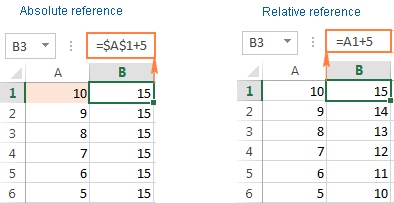Note. Though we have been saying that an absolute reference in Excel never changes, in fact it does change when you add or remove rows and/or columns in your worksheet, and this changes the location of the referenced cell. In the above example, if we insert a new row at the top of the worksheet, Excel is smart enough to adjust the formula to reflect that change: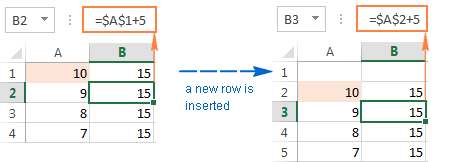In real worksheets, it's a very rare case when you'd use only absolute references in your Excel formula. However, there are a lot of tasks that require using both absolute and relative references, as demonstrated in the following examples.

## Using relative and absolute cell references in one formula

Quite often you may need a formula where some cell references are adjusted for the columns and rows where the formula is copied, while others remain fixed on specific cells. In other words, you have to use relative and absolute cell references in a single formula.

#### Example 1. Relative and absolute cell references for calculating numbers

In our previous example with USD and EUR prices, you may not want to hardcode the exchange rate in the formula. Instead, you can enter that number in some cell, say C1, and fix that cell reference in the formula by using the dollar sign (\$) like shown in the following screenshot: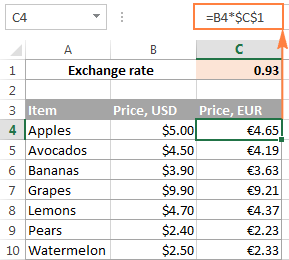In this formula (B4*\$C\$1), there are two cell reference types:

• B4 - relative cell reference that is adjusted for each row, and
• \$C\$1 - absolute cell reference that never changes no matter where the formula is copied.

An advantage of this approach is that your users can calculate EUR prices based on a variable exchange rate without changing the formula. Once the conversion rate changes, all you have to do is to update the value in cell C1.

#### Example 2. Relative and absolute cell references for calculating dates

Another common use of absolute and relative cell references in a single formula is Calculating dates in Excel based on today's date.

Supposing you have a list of delivery dates in column B, and you input the current date in C1 by using the TODAY() function. What you want to know is in how many days each item ships, and you can calculate this out by using the following formula: `=B4-\$C\$1`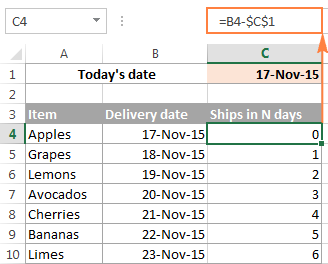And again, we use two reference types in the formula:

• Relative for the cell with the first delivery date (B4), because you want this cell reference to vary depending on the row where the formula resides.
• Absolute for the cell with today's date (\$C\$1), because you want this cell reference to remain constant.

Wrapping up, whenever you want to create an Excel static cell reference that always refers to the same cell, be sure to include the dollar sign (\$) in your formula to create an absolute reference in Excel.

## Excel mixed cell reference

A mixed cell reference in Excel is a reference where either the column letter or a row number is fixed. For example, \$A1 and A\$1 are mixed references. But what does each mean? It's very simple.

As you remember, an Excel absolute reference contains 2 dollar signs (\$) that lock both the column and the row. In a mixed cell reference, only one coordinate is fixed (absolute) and the other (relative) will change based on a relative position of the row or column:

• Absolute column and relative row, like \$A1. When a formula with this reference type is copied to other cells, the \$ sign in front of the column letter locks the reference to the specified column so that it never changes. The relative row reference, without the dollar sign, varies depending on the row to which the formula is copied.
• Relative column and absolute row, like A\$1. In this reference type, it's the row's reference that won't change, and the column's reference will.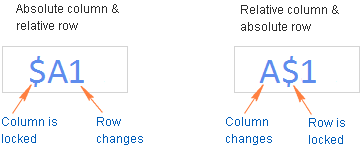Below you will find an example of using both mixed cell reference types that will hopefully make things easier to understand.

#### Using a mixed reference in Excel - formula example

For this example, we will be using our currency conversion table again. But this time, we won't limit ourselves only to the USD - EUR conversion.  What we are going to do is to convert the dollar prices to a number of other currencies, all with a single formula!

To begin with, let's enter the conversion rates in some row, say row 2, as shown in the screenshot below. And then, you write just one formula for the top-left cell (C5 in this example) to calculate the EUR price:

`=\$B5*C\$2`

Where \$B5 is the dollar price in the same row, and C\$2 is the USD - EUR conversion rate.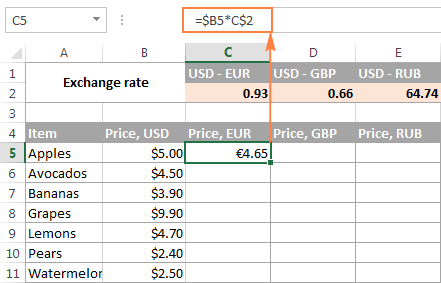And now, copy the formula down to other cells in column C, and after that auto-fill other columns with the same formula by dragging the fill handle. As the result, you will have 3 different price columns calculated correctly based on the corresponding exchange rate in row 2 in the same column. To verify this, select any cell in the table and view the formula in the formula bar.

For example, let's select cell D7 (in the GBP column). What we see here is the formula `=\$B7*D\$2` that takes a USD price in B7 and multiplies it by the value in D2, which is the USD-GBP conversion rate, just what the doctor ordered :)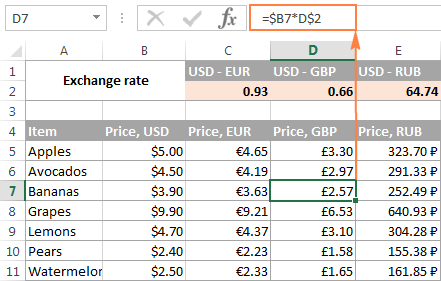And now, let's understand how it comes that Excel exactly knows which price to take and which exchange rate to multiply it by. As you may have guessed, it's the mixed cell references that do the trick (\$B5*C\$2).

• \$B5 - absolute column and relative row. Here you add the dollar sign (\$) only before the column letter to anchor the reference to column A, so Excel always uses the original USD prices for all conversions. The row reference (without the \$ sign) is not locked because you want to calculate the prices for each row individually.
• C\$2 - relative column and absolute row. Because all the exchange rates reside in row 2, you lock the row reference by putting the dollar sign (\$) in front of the row number. And now, no matter what row you copy the formula to, Excel will always look for the exchange rate in row 2. And because the column reference is relative (without \$ sign), it will get adjusted for the column to which the formula is copied.

## How to reference an entire column or row in Excel

When you are working with an Excel worksheet that has a variable number of rows, you may want to refer to all of the cells within a specific column. To reference the whole column, just type a column letter twice and a colon in between, for example A:A.

#### A whole-column reference

As well as cell references, an entire column reference can be absolute and relative, for example:

• Absolute column reference, like \$A:\$A
• Relative column reference, like A:A

And again, you use the dollar sign (\$) in an absolute column reference to lock it to a certain column, for the entire-column reference not to change when you copy a formula to other cells.

A relative column reference will change when the formula is copied or moved to other columns and will remain intact when you copy the formula to other cells within the same column.

#### A whole-row reference

To refer to the entire row, you use the same approach except that you type row numbers instead of column letters:

• Absolute row reference, like \$1:\$1
• Relative row reference, like 1:1

In theory, you can also create a mixed entire-column reference or mixed entire-row reference, like \$A:A or \$1:1, respectively. I say "in theory", because I cannot think of any practical application of such references, though Example 4 proves that formulas with such references work exactly as they are supposed to.

#### Example 1. Excel entire-column reference (absolute and relative)

Supposing you have some numbers in column B and you want to find out their total and average. The problem is that new rows are added to the table every week, so writing a usual SUM() or AVERAGE() formula for a fixed range of cells is not the way to go. Instead, you can reference the entire column B:

`=SUM(\$B:\$B)` - use the dollar sign (\$) to make an absolute whole-column reference that locks the formula to column B.

`=SUM(B:B)` - write the formula with no \$ to make a relative whole-column reference that will get changed as you copy the formula to other columns.

Tip. When writing the formula, click the column letter to have the entire-column reference added to the formula. As is the case with cell references, Excel inserts a relative reference (with no \$ sign) by default: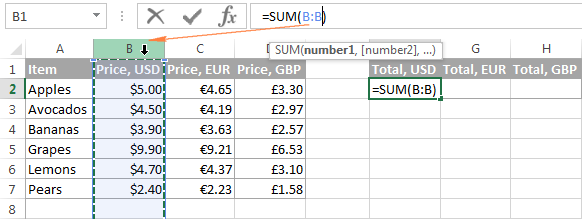In the same fashion, we write a formula to calculate the average price in the whole column B:

`=AVERAGE(B:B)`

In this example, we are using a relative entire-column reference, so our formula gets adjusted properly when we copy it to other columns: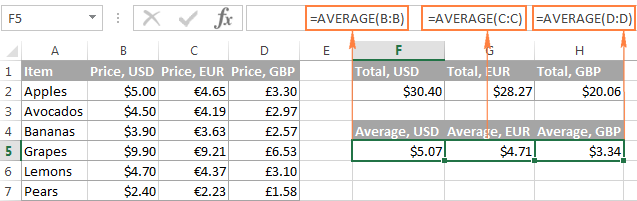Note. When using an entire-column reference in your Excel formulas, never input the formula anywhere within the same column. For example, it might seem like a good idea to enter the formula =SUM(B:B) in one of the empty bottom-most cells in column B to have the total at the end of the same column. Don't do this! This would create a so-called circular reference and the formula would return 0.

#### Example 2. Excel entire-row reference (absolute and relative)

If the data in your Excel sheet is organized in rows rather than columns, then you can reference an entire row in your formula. For example, this is how we can calculate an average price in row 2:

`=AVERAGE(\$2:\$2)` - an absolute whole-row reference is locked to a specific row by using the dollar sign (\$).

`=AVERAGE(2:2)` - a relative whole-row reference will change when the formula is copied to other rows.

In this example, we need a relative entire-row reference because we have 3 rows of data and we want to calculate an average in each row by copying the same formula: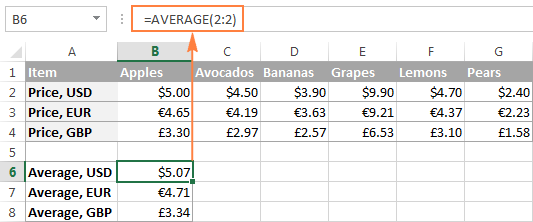#### Example 3. How to refer to an entire column excluding the first few rows

This is a very topical problem, because quite often the first few row in a worksheet contain some introductory clause or explanatory information and you don't want to include them in your calculations. Regrettably, Excel does not allow references like B5:B that would include all the rows in column B beginning with row 5. If you try adding such a reference, your formula will most likely return the #NAME error.

Instead, you can specify a maximum row, so that your reference includes all possible rows in a given column. In Excel 2016, 2013, 2010, and 2007, a maximum is 1,048,576 rows and 16,384 columns. Earlier Excel versions have a row maximum of 65,536 and column maximum of 256.

So, to find an average for each price column in the below table (columns B through D), you enter the following formula in cell F2, and then copy it to cells G2 and H2:

`=AVERAGE(B5:B1048576)`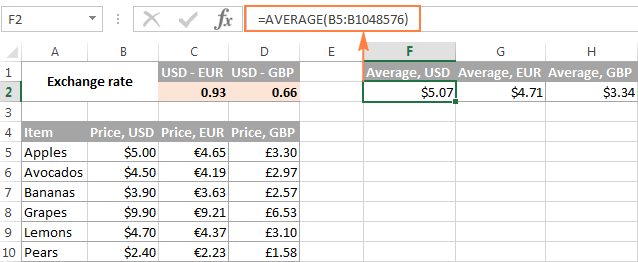If you are using the SUM function, you can also subtract the rows you want to exclude:

`=SUM(B:B)-SUM(B1:B4)`

#### Example 4. Using a mixed entire-column reference in Excel

As I mentioned a few paragraphs before, you can also make a mixed entire-column or entire-row reference in Excel:

• Mixed column reference, like \$A:A
• Mixed row reference, like \$1:1

Now, let's see what happens when you copy a formula with such references to other cells. Supposing you input the formula `=SUM(\$B:B)` in some cell, F2 in this example. When you copy the formula to the adjacent right-hand cell (G2), it changes to `=SUM(\$B:C)` because the first B is fixed with the \$ sign, while the second isn't. As the result, the formula will add up all the numbers in columns B and C. Not sure if this has any practical value, but you may want to know how it works: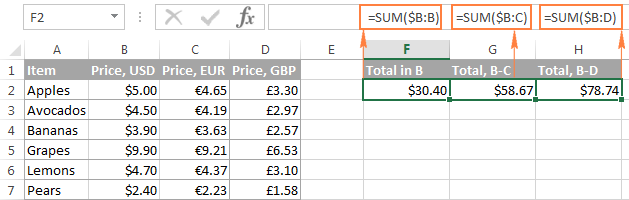A word of caution! Don't use too many entire column/row references in a worksheet because they may slow down your Excel.

## How to switch between absolute, relative, and mixed references (F4 key)

When you write an Excel formula, \$ sign can of course be typed manually to change a relative cell reference to absolute or mixed. Or, you can hit the F4 key to speed things up. For the F4 shortcut to work, you have to be in formula edit mode:

1. Select the cell with the formula.
2. Enter Edit mode by pressing the F2 key, or double-click the cell.
3. Select the cell reference you want to change.
4. Press F4 to toggle between four cell reference types.

If you've selected a relative cell reference with no \$ sign, like A1, repeatedly hitting the F4 key toggles between an absolute reference with both dollar signs like \$A\$1, absolute row A\$1, absolute column \$A1, and then back to the relative reference A1.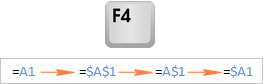Note. If you press F4 without selecting any cell reference, the reference to the left of the mouse pointer will get selected automatically and changed to another reference type.

I hope now you fully understand what relative and absolute cell references are, and an Excel formula with \$ signs is no longer a mystery. In the next few articles, we will continue learning various aspects of Excel cell references such as referencing another worksheet, 3d reference, structured reference, circular reference, and so on. In the meantime, I thank you for reading and hope to see you on our blog next week!

### 62 Responses to "Why use dollar sign (\$) in Excel formulas - absolute and relative cell references"

1.AK Pandey says:

Hi Sirji,
I want to find out the highest marks in the entire sheet for each code separately
formula put in All marks columns

code marks code marks code marks code marks code marks
301 90 30 35 85 99 65 45 301 76
40 92 41 65 30 85 41 36 37 85
41 85 301 22 41 92 55 85 55 70
30 35 48 25 301 45 40 77 30 55

•pas says:

First you type the list in the following manner or you can paste it in this style by your origin table, because of computers works then when you give it (computer) perfect quary status.
code marks
301 90
40 92
41 85
30 35
30 35
41 65
301 22
48 25
85 99
30 85
41 92
301 45
65 45
41 36
55 85
40 77
301 76
37 85
55 70
30 55
After it select this table and press Ctrl + L (it helps you to convert it in table style format), then go to design menu (which would be appear after fomat apply by press Ctrl+L). There is in design menu a sub menu tool in which first tool is "Summarize with PivotTable", click it and by default it give you, your data result in next sheet and that sheet would be create by "Pivot Table". Now Press Ok. Step 1 - Drag "Code" into "Row Label". Step 2 - Drag "Marks" into "Values" (when you draged Marks into Value it would be convert into "sum of Marks") Step 3- Click on "Sum of Marks", then click on value field setting, now click on max (instead of "sum"). Here you are find your result that is following :-
30 85
37 85
40 92
41 92
48 25
55 85
65 45
85 99
301 90

2.Gilberto says:

Nice report. Congratulations !
Sometimes I use long formulas that include absolute cell references associated with relative cell references. When copying such formulas for dozens of novel columns, I have to individually change the absolute cell column identification of each formula, which is time consuming. Is there any general way to push Excel to assume that the absolute cell reference of a given formula corresponds to that of the column the formula is located?
Thankyou.
Best regards,
Dr. Gilberto Xavier, University of São Paulo, Brasil

•Svetlana Cheusheva (Ablebits.com Team) says:

Hello Gilberto,

Nope, there is no such way. This is the most essential difference between the relative and absolute references. An absolute reference is locked to the particular column and row, while a relative reference adjusts to the column and row where the formula is located.

It's difficult to give any advice without seeing your data, but maybe it would make sense to use absolute row and relative column references in your formula for the column reference to change depending on the column to which the formula is copied.

3.ABDUL MOIN says:

I AM GOING TO PREPARE A WORKBOOK IN EXCEL HAVING MANY EXCEL SHEETS OF VARIOUS STOCK IN AND OUT RECORD AND NET FIGURE IN LAST COLUMN OF THE SHEET WHICH CHANGE THE FIGURE IN NEXT CELL OF THE COLUMN WHEN A UPDATE IS DONE IN THE SHEET.
I AM ALSO GOING TO MAKE A SUMMURY SHEET IN THE SAME WORK BOOK HAVING HORIZONTALY NAME OF EACH ITEM OR EACH MATERIAL AND NEED TO SHOW THE NET RESULT OF EACH MATERIAL SHEET IN FRONT OF EACH ITEM WHEN EVER CHANGE IS MADE IN ANY OF THE MATERIAL SHEET.

KINDLY ADVISE ME THE FORMULA TO BE PUT IN A CELL AGAINST THE EACH ITEM IN SUMMURY SHEET TO PICK THE RESULT FROM THE RELATIVE SHEETS.

THANKS AND REGARDS

4.suchi says:

what is the short cut of \$ sign

•Svetlana Cheusheva (Ablebits.com Team) says:

Hi Suchi,

With the cursor in the reference, or immediately before or after it, you can use the function key F4 to toggle through \$A\$1 -> \$A1 -> A\$1 and back to A1.

Please see How to switch between absolute, relative, and mixed references for full details.

•Somnath says:

Dear Svetlana,

5.jyoti srivastava says:

what is featurs of cell reference and advantage and diadvantage of cell reference..plz give me ans..as soon as

6.Mike says:

Very excellent article. Well understood. I'm very impressed. Thanks a million

7.Dona says:

Just wanted to thank you so much for the straightforward explanation on the cell references. It now makes total sense thanks to you!

8.Simon says:

Is there a way to change the direction of formulas autofill - so once I copy a formula downwards in a column it'll gather data from the consecutive cell to the right (in the adjacent column) and not the consecutive cell down (in the same column)? In short i need to transpose a formula.

9.Masoud says:

appreciate it. very useful.

10.chrisp909 says:

Thank you for this. I've been using excel formulas / macros for years. I even use absolute/relative references fairly often. For whatever reason they've always confused me and i get them switched around. Your article crystallized in my mind information I should have already had. It's embarrassing really but kudos for a well written article. :)

•Svetlana Cheusheva (Ablebits.com Team) says:

Thank you, Chrisp. I am really pleased to know that my article has proved helpful.

11.Gary says:

What happens to "\$"s that are not part of addresses? Any problems?

12.Deba says:

Hi Svetlana! thank you for an excellent article! I have a work sheet that I have all the equipment that needs yearly certification. if I assign cell G19 to be the date of last certification and H19 to be next due certification (which a year from the last certification)what would be my formula for H19. and also if it is less than a year the cell should be green and if it is over a year the cell should turn Red and if is within 10month of the certification it should turn yellow. the formula should be for entire H Column. I hope it make sense to you.

13.Mahdi says:

Thank you
You are a life saver
It was good to know how to use f4 toggling to a non \$ reference

14.Balbir Singh says:

Realy thanks to you

15.123 says:

hi
it helped me very much but i have a question how do you make other cells fixed.

16.Benjamin says:

well written! This thing has confused me for many many years(even as an Engineer!) but you made it look sooo simple. Thanks

17.Jim Petroulas says:

Dont know if i can solve the problem i have with absolute cell reference. The problem is if i have a row of data (and i want it to refer to a column of data) even if i make a two cell formula to get back data from column, after dragging them both the form ends up bringing back row data. Thanks

18.Chandler says:

How do you incorporate the absolute cell reference in a date? Instructions say to use an absolute reference to the date in cell C20 and the date is 10/14/2016 but nothing is telling me how to use the absolute cell reference with that

19.Randy says:

I am using Excel online to create a checkbook. I have tried to use absolute reference formulas, but the system doesn't save the formula. Anyone know if this can be done online

20.Ankur Kabra says:

How can i switch between relative at the one time shot in whole excel sheet?

21.Redempter Ndegwa says:

thank you for your wonderful services

22.Jean-Pierre says:

How can I change Excel to default to relative cell references (instead of absoulte references it currently defaults to)?

23.Amgad says:

Hello Sir,
\$ fix column E1, E2, E3,
If i want to fix row E1, F1, G1.
Thank you

24.Shayyan Ali says:

Thank you very much for this informative post ,ALLAH solved my problem from this post.

•Kek says:

it was Jesus boi. But don't be afraid - everything is good. God i love. Peace.

25.Jo Bassler says:

How do I make absolute cell references to items in a named range?

For example: create a named range, called Phone using A1:a128, make sure the cell references are absolute.

•Svetlana Cheusheva (Ablebits.com Team) says:

Hi Jo,

Named range references are absolute by their nature. In other words, no matter where you use or copy a formula referencing your "Phone" range, it will always refer to cells A1:A128.

26.Fred Zarse says:

I am printing coupons for a monthly buyer. I want to print June in the first coupon, and have Excel print the next months (July, Aug, Sept) in the next 12 coupons. ??
Windows 10

27.Ignacio says:

What is the equivalent of \$ a table? In a table it shows like table[X]

•Svetlana Cheusheva (Ablebits.com Team) says:

Hi Ignacio,

When you create a table, Excel automatically assigns a name to it, as well as to each column in the table. Those names appear in a formula if you select the cell references in the table instead of entering them manually. And that combination of table and column names is called a structured reference.

To make a structured references absolute, you need to duplicate the reference as if it were a range of multiple columns. For example:

Table1[[Column1]:[Column1]] will be locked to Columnn1.

Please note that to copy such formulas, you must drag them across columns using the mouse.

28.Didar Karim says:

Very nicely articulated the use of \$ in Excel and covered every aspect. Thanks a million

29.Musa Enocy says:

30.Heather Hanley says:

Your articles are excellent and I usually find a fix to an issue. If I am bringing in the contents from a cell in another spreadsheet, it makes it absolute. if I want to drag that down it takes the absolute with it. If I remove the absolute I can then drag it down and get what I want - relative numbers but, I then want to make them absolute. Is there a quick way of making them all absolute?

31.Ali says:

Hi

i am working on 2 sheets in a workbook, SheetA and SheetB
i am using cell reference from Sheet B in Sheet A.

I want something like below when i drag down:
A1 of SheetA is from D1 of SheetB.
A2 of SheetA should be D3 of SheetB.
A3 of SheetA should be D5 of SheetB.
A4 of SheetA should be D7 of SheetB.

32.asad says:

got stuck in a formula in my thesis.now i got a clear understanding about \$ sign.well written.actually description with figures helped a lot.thanks miss

33.Merisha says:

Thank you so much! Very helpful

34.Navneet says:

I have a column in one sheet where I want data to be populated. In my other sheet I have the data that is to be populated which is plotted in one row.

What I want is that when I pull down the cell formula from A2 to A3 from the first sheet it should pick up the value in the next cell in the next column in in the same row in the second sheet ie from A2 to B2.

Is it possible?

•Gennady Terekhov (Ablebits.com Team) says:

Hello,

=INDEX(Sheet2!\$2:\$2,1,ROW(A1))

35.Karl says:

Thank you so much for your excellent tutorial. Can you tell me how to make a formula that references the output of a previously calculated formula and keeps changing row by row? I want to make a budget with a running balance that changes on each new row, sort of like C3=C2-B3, followed by C4=C3-B4. Many thanks.

36.Karl says:

No need to reply. I just played with what I learned from your tutorial and made the formula straight away! Thanks again!

37.Karl says:

In case others want to know the solution, it's =\$D4+\$B5-\$C5 where column D is income, column B is expense, and column C is current balance. The only problem is that when pasting the formula all that way down column C, it lists the value in the last row of data entered all the way. I'm sure there's a solution for this and will search for it.

38.anwer says:

dear,
hi i created one sheet, Item Name,Pack Size, price of items, different parties Sales, Bonus, Sales Bonus, Sales Bonus, Sales Bonus, Sales Bonus, Sales Bonus, Sales Bonus, Sales Bonus, for the whole month same sales and Bonus columns a daily basis will be 8 orders i want total sales items and bonus sepreated kindly help me in this problem

39.KitPal says:

In sheet 1:
Column A is ‘ITEM’, column B is ‘Balance Count’
Row A2 is RR1
Row A3 is RR2
.
.
.
Row An is RRn

In sheet 2:
Column A is ‘ITEM’, Column B is ‘Inward Qty’, Column C is ‘Issued Qty’, Column D is ‘Date’ and Column E is ‘Balance Count’.

Row A2 is RR1
Equation for ‘Balance Count' E2 is =(B2-C2)
Equation for ‘Balance Count' E3 to En is =IF(AND(ISBLANK(B3),ISBLANK(C3))," ",(E2+B3-C3))

My Question is
Balance Count B2 in sheet1 should be updated whenever the last Balance Count against RR1 (E2:En) is modified in sheet 2

40.Helpneeded says:

Hi all, need a quick help here!
Supposedly I understand excel default is relative cell reference. But when I copy over a formula, it's not updating the references of the new columns/rows.

Eg. formula =[@[Dec 17]]*(1+E3)

Note: @[Dec 17] is referencing to a cell under the header of "Dec 17".
When the cell is selected, the formula shows @[Dec 17] instead of the cell location G2 (which is what I want).

How do I fix it?

•Chris says:

I think that's conditional formatting

41.Maxime says:

Thank you very much for this review.

Your explanations are simple and straight to the point. You make a great job in making them applicable, and the cases that you use as examples just sink the concepts in.

So thanks once more.

42.Alex Khan says:

Hi
What does this mean in Excel

=VLOOKUP(B12,'file1.xlsb]Sheet1'!\$A:\$J,10,FALSE

Especially !\$A:\$J,10,FALSE

•Doug says:

Alex:
This tells Excel to lookup the value in cell B12 in the file1 xlsb workbook that matches the value on Sheet 1 in the range A:J, is in the 10th column from the left and it doesn't have to be an exact match.
The exclamation point signifies the Sheet1 is a sheet in the file1.xlsb workbook.

•Alex says:

Thanks so much for this explaination.

Can tyhis be simplified at all ? as the file1.xlsb only has one sheet in there anyway ?

Cheers
Alex

43.senthil says:

The strike through functions is not worked when referenced with the previous tab. Can you help me to clear this please.

44.Blake EVES says:

Hi Svetlana, I have an interesting dilema and hopeing you can point me in the right direction. In column A, i have a list of various items, lets call them Cars and Trucks. In column B I have a list of hours associated with each item in Column A. I now want to do a sum of hours based on items in Column A...pretty stratight forward with something like =SUMIF(\$A\$1:\$A\$1000,"Car",\$B\$1:\$B\$1000). But now I want to use another Cell to determine the row number to use in my range...so something like \$A:\$(value obtained from input) Any ideas?

45.mohammed aadil says:

46.Zia ur Rehman says:

Excellent working
zia

47.SHAKEEL says:

WHICH OF THE FOLLOWING FORMULAS CORRECTLY ANCHORS ONLY THE ROW IN CELL A1?
1. A1
2.A \$1
3.\$A \$ 1
4.\$A1

48.SHAKEEL says:

WHICH OF THE FOLLOWING FORMULAS PROPERLY PRODUCES THE STRING "INCOME STATEMENT"?

49.junkyard says:

Hi. First thanks for ur excellent lesson here.
I have a workbook with a few sheets, one sheet is called "day to day" where i enter all my expenses, each expense is entered separately on there own row (like a bank register). And each column is meant for there thing, for example: in column A i write the date when the expense was, in column B i write the amount, then in column D i write the type of expense, like "insurance", "gas", etc..
Now on a different sheet i have a table that calculates the total of each type of expense, every year, it looks something like this:
A B C
1 EXPENSSES 2017 2018
2 Insurance \$ - \$ -
3 Maintenance \$ - \$ -

I entered in cell 'C1' this,
=SUMIFS('Day To Day'!B:B,'Day To Day'!A:A,">="&DATE(2018, 1, 1),'Day To Day'!A:A,"<"&DATE(2019, 1, 1),'Day To Day'!D:D,"Insurance")
it gives me the total how much i paid for insurance in the year of 2018.

Now when i want to add to the table for the next year, cell D2 should be for 2019, and i try to move with the fill handle (the small cross) from C2 to D2, the date doesn't change, even though i didn't put a \$ sign by the date, so what correction do i need to get this done?

60+ professional tools for Excel